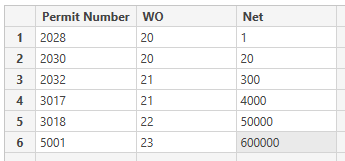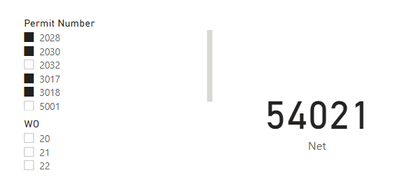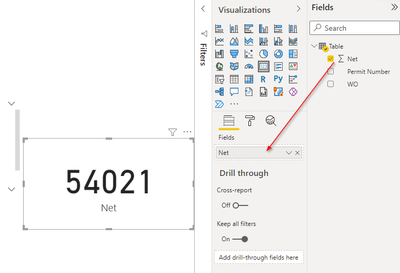cancel
Showing results for
Did you mean:

Fabric is Generally Available. Browse Fabric Presentations. Work towards your Fabric certification with the Cloud Skills Challenge.Frequent Visitor

## Dax to Calculate; Filter Multiple Columns with And

Hello!

I have a table with the following columns: Permit Number, WOs, Net and I need to create a filter for my Card visual to get the correct results. I've tried to write numerous measures and can't get anything to work correctly. I am also not very good at this so I am sure I am doing something wrong.

I am trying to get the Total of the Net with the following filters applied: A filter to return the Total Net for Permit Number 5001 and only the total net for WO's 20, 21, and 22. And a filter to return the total net for Permit Numbers 2030, 2032, 3016, and 3017. Is this possible?

Thank you so much in advance!

2 ACCEPTED SOLUTIONSSuper User

@emae613
As said. This is no problem. The code can be modified as follows:

``````Measure1 =
VAR T1 =
FILTER ( ALL ( 'Table' ), 'Table'[Permit Number] = 5001 )
VAR T2 =
FILTER ( ALL ( 'Table' ), 'Table'[WOs] IN { "20", "21", "22" } )
VAR T3 =
UNION ( T1, T2 )
VAR Result =
SUMX ( T3, 'Table'[Net] )
RETURN
Result``````
``````Measure2 =
VAR T1 =
FILTER ( ALL ( 'Table' ), 'Table'[Permit Number] IN { 2030, 2032, 3016, 3017 } )
VAR Result =
SUMX ( T1, 'Table'[Net] )
RETURN
Result``````

Please le me know if you need help.Super User

Yes you can create a new measure
Mearure3 = {Measur1] + [Measure2]
Then use it in your Card Visual

12 REPLIES 12Super User

Hi @emae613
Yes it is possible. If I fully understand your requirement then you can use the following codes (Make sure the columns Permit Number & WOs have integer data type not text)

``````Measure1 =
VAR T1 =
FILTER ( ALL ( 'Table' ), 'Table'[Permit Number] = 5001 )
VAR T2 =
FILTER ( ALL ( 'Table' ), 'Table'[WOs] IN { 20, 21, 22 } )
VAR T3 =
UNION ( T1, T2 )
VAR Result =
SUMX ( T3, 'Table'[Net] )
RETURN
Result``````
``````Measure2 =
VAR T1 =
FILTER ( ALL ( 'Table' ), 'Table'[Permit Number] IN { 2030, 2032, 3016, 3017 } )
VAR Result =
SUMX ( T1, 'Table'[Net] )
RETURN
Result``````

Please let me know if you face any trouble applying above or in case I misunderstood your requirement. Thanks and have a great day!Frequent Visitor

Thank you so much for your solution on this. Unfortunately, my WO column is a combination of Text and Whole Numbers so it won't let me convert it.Super User

@emae613
As said. This is no problem. The code can be modified as follows:

``````Measure1 =
VAR T1 =
FILTER ( ALL ( 'Table' ), 'Table'[Permit Number] = 5001 )
VAR T2 =
FILTER ( ALL ( 'Table' ), 'Table'[WOs] IN { "20", "21", "22" } )
VAR T3 =
UNION ( T1, T2 )
VAR Result =
SUMX ( T3, 'Table'[Net] )
RETURN
Result``````
``````Measure2 =
VAR T1 =
FILTER ( ALL ( 'Table' ), 'Table'[Permit Number] IN { 2030, 2032, 3016, 3017 } )
VAR Result =
SUMX ( T1, 'Table'[Net] )
RETURN
Result``````

Please le me know if you need help.Frequent Visitor

So this is giving me the correct result:

Measure 2 =
VAR T1 =
Filter (ALL('RF21 Master Dose File'), 'RF21 Master Dose File'[RWP_NUMBER] IN { 222032, 223016, 223017, 223032} )
VAR Result =
Sumx (T1, 'RF21 Master Dose File'[NET_DOSE_REM])
Return
Result

Then I have this measure that is giving me the correct result:
Calculate(
[NET_DOSE_REM],
KEEPFILTERS(
'RF21 Master Dose File'[RWP_NUMBER] = 225001 &&
'RF21 Master Dose File'[WORK_REQUEST_NUMBER] in {"64436091","64436532","55571027"})
)

If there is a way to combine these 2 measures it would be the perfect solution. Thanks!Super User

What do you mean by "combine"? Add their values together?Frequent Visitor

Sorry about that. Yes, I need to add the values together so I can put the total into one card.Super User

Yes you can create a new measure
Mearure3 = {Measur1] + [Measure2]
Then use it in your Card VisualFrequent Visitor

Everything is working perfect! Thank you so much!Frequent Visitor

Thank you so much! Measure 1 doesn't seem to be giving me the correct total for the data. Measure 2 worked and is giving me the correct total for the data. However, is there a way to combine the 2 measures? I need both of the measures on one card. Measure 2 is giving me the sum of all the net for the permits but it is missing the data associated with permit 5001 and the 3 associated work orders. Is there a way to fix measure 1 and apply both measures to the card?Super User

@emae613
The code for measure one actually sums up the Net values for all records that do not include permit No. 5001 and then computes (separately) the Net values for all records that have WO's code either 20, 21 or 22. Then it sums up both values together. If this is not what you need, please try to expain to me exactly waht your requirement is.
Thank you!Super User

No problem. Just wrap the values inside the curly brackets with double quotes ""Anonymous
Not applicable

Hi @emae613 ,

Maybe you can provide some sample data to make it easier for other people to solve. This is my sample dataIf your Permit Number and WO are whole numbers, you can just use the slider in the slicer to choose the filters as belowIf the Permit Number and WO are text, then you can hold CTRL when choosing the filter you desired as belowOwh ya, for the card visual I just drag the Sum of Net into the field. No measure needed.Not sure if this is what you desired.Announcements#### Power BI Monthly Update - November 2023

Check out the November 2023 Power BI update to learn about new features.#### Fabric Community News unified experience

Read the latest Fabric Community announcements, including updates on Power BI, Synapse, Data Factory and Data Activator.#### The largest Power BI and Fabric virtual conference

130+ sessions, 130+ speakers, Product managers, MVPs, and experts. All about Power BI and Fabric. Attend online or watch the recordings.Top Solution Authors
Top Kudoed Authors
Users online (3,293)